Click to Chat

1800-1023-196

+91-120-4616500

CART 0

• 0

MY CART (5)

Use Coupon: CART20 and get 20% off on all online Study Material

ITEM
DETAILS
MRP
DISCOUNT
FINAL PRICE
Total Price: Rs.

There are no items in this cart.
Continue Shopping• Complete JEE Main/Advanced Course and Test Series
• OFFERED PRICE: Rs. 15,900
• View Details

```Chapter 1: Fractions Exercise – 2.1

Question: 1

Compare the following fractions by using the symbol > or < or =;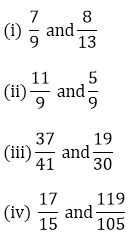Solution:

(i) We have,Taking the LCM of 9 and 13, we get,

9 x 13 = 117

Now, we convert the given fractions to equivalent fractions by making the denominators 117,As we know, 91 > 72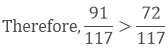Hence, 79 > 813

(ii)  We have,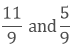The given fractions are equivalent fractions as the denominators are equal,

And we know that, 11 > 5

Therefore, 119 > 59

(iii)  We have,Taking the LCM of 41 and 30, we get,

41 x 30 = 1230

Now, we convert the given fractions to equivalent fractions by making the denominators 1230,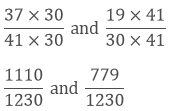Now, we clearly know 1110 > 779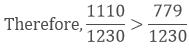Hence, 3741 > 1930

(iv) We have,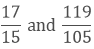Taking the LCM of 15 and 105, we get,

5 x 3 x 7 = 105

Now, we convert the given fractions to equivalent fractions by making the denominators 105,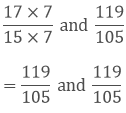Question: 2

Arrange the following fractions in ascending order: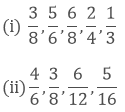Solution:

(i)  We have,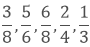Taking the LCM of 8, 6, 8, 4 and 3, we get,

2 x 4 x 3 = 24

Now, we convert the given fractions to equivalent fractions by making the denominators 24,We know that, 8 < 9 < 12 < 18 < 20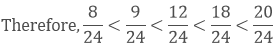Hence, 13 < 38 < 24 < 68 < 56

(ii) We have,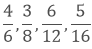Taking the LCM of 6, 8, 12 and 16, we get,

2 x 2 x 2 x 2 x 3 = 48

Now, we convert the given fractions to equivalent fractions by making the denominators 48,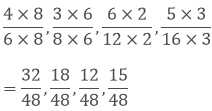We know that, 12 < 15 < 18 < 32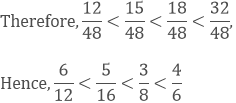Question: 3

Arrange the following fractions in descending order: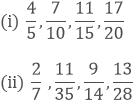Solution:

(i)  We have,Taking the LCM of 5, 10, 15 and 20, we get,

5 x 2 x 2 x 3 = 60

Now, we convert the given fractions to equivalent fractions by making the denominators 48As we know 51 > 48 > 44 > 42(ii) We have,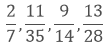Taking the LCM of 7, 35, 14 and 28, we get,

7 x 5 x 2 x 2 = 140

Now, we convert the given fractions to equivalent fractions by making the denominators 140As we know 40 > 44 > 65 > 90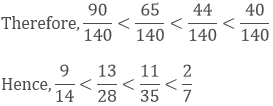Question: 4

Write the equivalent fractions of 3/5

Solution:

Multiplying or dividing both the numerator and denominator by the same number,

so that the fraction keeps its value.

So the equivalent fractions of 3/5 areare the five equivalent fractions of 3/5

Question: 5

Find the sum: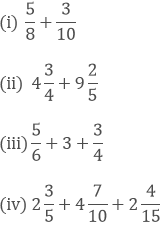Solution:

(i)  We have,Taking the LCM of 8 and 10, we get,

2 x 4 x 5 = 40

Now, we convert the given fractions to equivalent fractions by making the denominators 40(ii) We have,Taking out the LCM of 4 and 5, we get,

4 x 5 = 20

Now, we convert the given fractions to equivalent fractions by making the denominators 20(iii)  We have,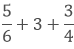Taking out the LCM of 6 and 4, we get,

2 x 2 x 3 = 12

Now, we convert the given fractions to equivalent fractions by making the denominators 12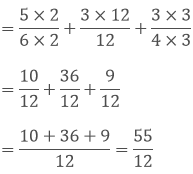(iv) We have,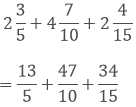Taking out the LCM of 5, 10 and 15, we get,

5 x 2 x 3 = 30

Now, we convert the given fractions to equivalent fractions by making the denominators 30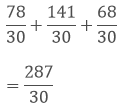Question: 6

Find the difference ofSolution:

(i)  We have,Taking out the LCM of 24 and 16, we get,

2 x 2 x 2 x 2 x 3 = 48

Now, we convert the given fractions to equivalent fractions by making the denominators 48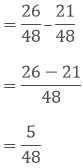(ii)  We have, 6 and 23/3

The difference between 6 and 23/3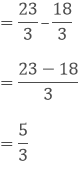(iii)  We have,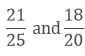Taking out the LCM of 25 and 20, we get,

5 x 5 x 4 = 100

Now, we convert the given fractions to equivalent fractions by making the denominators 100The difference between both the fractions are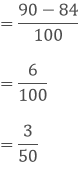(iv) We have,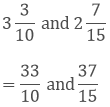Taking out the LCM of 10 and 15, we get,

2 x 3 x 5 = 30

Now, we convert the given fractions to equivalent fractions by making the denominators 30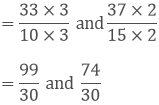The difference between both the fractions areQuestion: 7

Find the difference: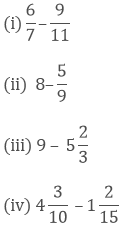Solution:

(i)  We have,Taking out the LCM of 7 and 11, we get,

7 x 11 = 77

Now, we convert the given fractions to equivalent fractions by making the denominators 77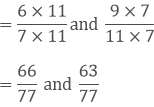The difference between both the fractions are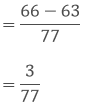(ii)  We have,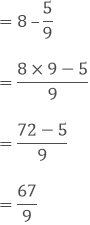(iii) We have,(iv) We have,Taking out the LCM of 10 and 15, we get,

2 x 3 x 5 = 30

Now, we convert the given fractions to equivalent fractions by making the denominators 30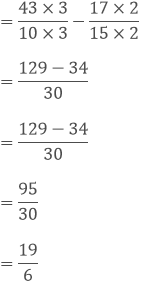Question: 8

Simplify: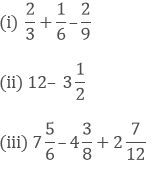Solution:

(i) We have,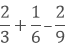Taking out the LCM of 3, 6 and 9, we get,

3 x 3 x 2 = 18

Now, we convert the given fractions to equivalent fractions by making the denominators 18, we get,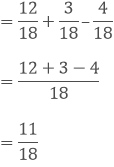(ii)  We have,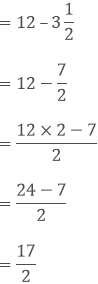(iii) We have,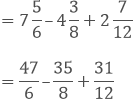Taking out the LCM of 6, 8 and 12, we get,

2 x 2 x 2 x 3 = 48

Now, we convert the given fractions to equivalent fractions by making the denominators 48, we get,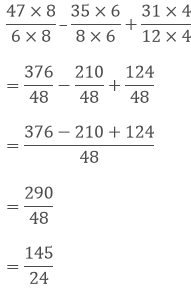Question: 9

What should be added toto get 12?

Solution:

We have,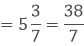Let x be the number added to 38/7 to get 12

Therefore,

x + 387 = 12Question: 10

What should be added to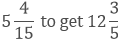?

Solution:Let x be the number added to 79/15 to get 63/5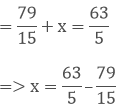Taking out the LCM of 5 and 15, we get,

3 x 5 = 15

Now, we convert the given fractions to equivalent fractions by making the denominators 15, we get,Question: 11

Suman studies for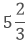hours daily. She devotes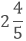hours of her time for science and mathematics. How much time does she devote for other subjects?

Solution:

Given,

Suman studies forhours daily

She devoteshours of her time for science and mathematics. Let x be time she devotes for other subjects.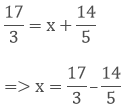Taking out the LCM of 3 and 5, we get,

3 x 5 = 15

Now, we convert the given fractions to equivalent fractions by making the denominators 48, we get,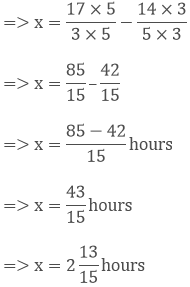Question: 12

A piece of wire of length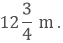If it is cut into two pieces in such a way that the length of one piece is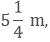what is the length of the other piece?

Solution:

A piece of wire of lengthone piece isLet the length of other piece be x m.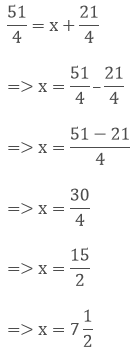Question: 13

A rectangular piece of paper is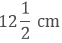long andwide. Find its perimeter?

Solution:

Given,

A rectangular piece of paper islong andwidePerimeter = 2(length + width)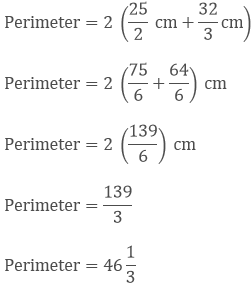Question: 14

In a “magic square”, the sum of numbers in each row, in each column and along the diagonal is same. Is this a “magic square”?

4/11
9/11
2/11

3/11
5/11
7/11

8/11
1/11
6/11

Solution:

Given,

4/11
9/11
2/11

3/11
5/11
7/11

8/11
1/11
6/11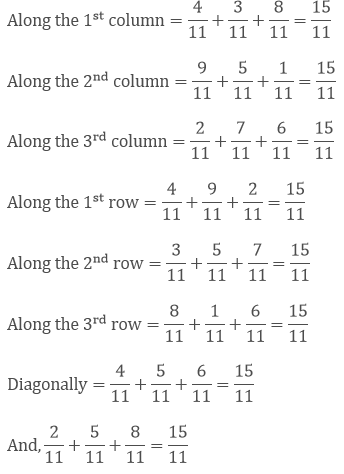Therefore, the sum of numbers in each row, in each column and along the diagonal is same and the sum is 15/11.

Question: 15

The cost of Mathematics book is Rs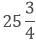and that of science book isWhich costs more and by how much?

Solution:

Given,

The cost of Mathematics book is Rsand that of science book isWe need to compare the cost of mathematics and science book,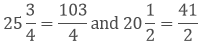Taking out the LCM of 4 and 2, we get,

2 x 2 = 4

Now, we convert the given fractions to equivalent fractions by making the denominators 4, we get

= 103/4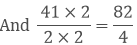As we know, 103 > 82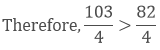Hence, the cost of mathematics book is more than that of the cost of the science book.

Question: 16

Provide the number in the box [] and also give its simplest form in each of the following:Solution: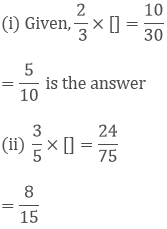```### Course Features

• 728 Video Lectures
• Revision Notes
• Previous Year Papers
• Mind Map
• Study Planner
• NCERT Solutions
• Discussion Forum
• Test paper with Video Solution